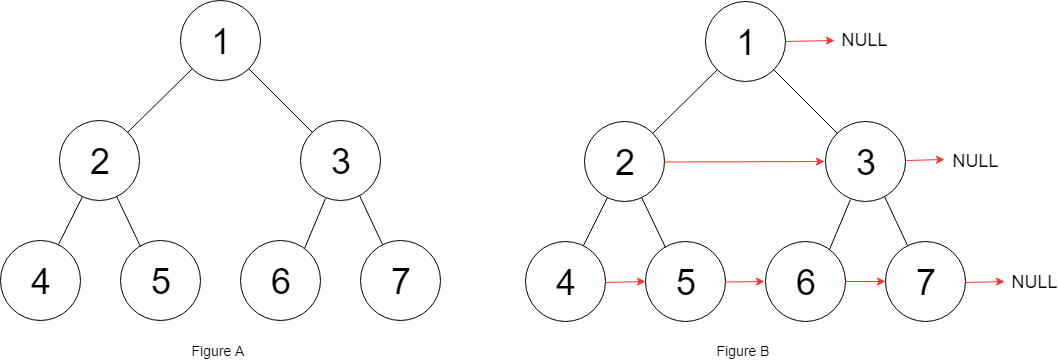# LeetCode – Populating Next Right Pointers in Each Node Solution

### 题目：

You are given a perfect binary tree where all leaves are on the same level, and every parent has two children. The binary tree has the following definition:

```struct Node {
int val;
Node *left;
Node *right;
Node *next;
}
```

Populate each next pointer to point to its next right node. If there is no next right node, the next pointer should be set to `NULL`.

Initially, all next pointers are set to `NULL`.

• You may only use constant extra space.
• Recursive approach is fine, you may assume implicit stack space does not count as extra space for this problem.

Example 1:Input:

``` root = [1,2,3,4,5,6,7]
```

Output:

``` [1,#,2,3,#,4,5,6,7,#]
```

Explanation:

```Given the above perfect binary tree (Figure A), your function should populate each next pointer to point to its next right node, just like in Figure B. The serialized output is in level order as connected by the next pointers, with '#' signifying the end of each level.
```

Constraints:

• The number of nodes in the given tree is less than `4096`.
• `-1000 <= node.val <= 1000`

### 实现：

由于是完全二叉树，所以有左，右子树同时存在，左子树next节点为对应的右子树； 右子树的next节点为其父节点的next节点的左子树 。 父节点next为空，则为空。

• 递归方式
``````
// Definition for a Node.
class Node {
public:
int val;
Node* left;
Node* right;
Node* next;

Node() : val(0), left(NULL), right(NULL), next(NULL) {}

Node(int _val) : val(_val), left(NULL), right(NULL), next(NULL) {}

Node(int _val, Node* _left, Node* _right, Node* _next)
: val(_val), left(_left), right(_right), next(_next) {}
};
*/

/* Recursive
class Solution {
public:
Node* connect(Node* root) {
//check and set next
if (root==NULL) {
return NULL;
}

if (root->left) {
root ->left -> next = root->right;
}

if (root->right) {
root ->right -> next = root->next ? root->next->left : NULL;
}
//left
connect(root->left);
//right
connect(root->right);

return root;
}
};
``````
• 水平遍历,  由于是完全二叉树， 水平遍历取出的节点的next节点为队列下一个节点， 对于最后一个节点 size-1进行特殊处理即可。
``````class Solution {
public:
Node* connect(Node* root) {
if (root == NULL) {
return NULL;
}
queue<Node *> q;
q.push(root);

while (!q.empty()) {
size_t  size = q.size();

for (size_t i=0; i<size; i++){
Node* node = q.front();
q.pop();
if (i != size-1)
node->next = q.front();
else
node->next = NULL;

if (node->left) {
q.push(node->left);
}
if (node->right) {
q.push(node->right);
}
}
}

return root;
}
};``````

• 其他解法： 思路是一样的是通过两层循环实现，使用两个指针，一个指针pre指向每层的第一个节点， 通过cur节点进行遍历， 其左子树next=cur->right, 其右子树next=cur->next->left。 完成本次遍历，重新设置每层第一个节点left，进行下一层遍历。
``````
class Solution {
public:
Node* connect(Node* root) {
if (root == NULL){
return root;
}
Node*  pre = root ;
Node*  cur = NULL;
while (pre->left){
cur = pre;
while (cur) {
cur->left->next = cur->right;
if (cur->next) {
cur->right->next = cur->next->left;
}
cur = cur->next;
}
pre = pre->left;
}

return root;
}
};``````